# Model Calibration

Edgar Ruiz

I am very excited to introduce work currently underway on the probably package.
We are looking to create early awareness and receive feedback from the community. That is why the enhancements discussed here are not yet on CRAN.

While the article is meant to introduce new package functionality, we also have the goal of introducing model calibration conceptually. We want to provide sufficient background for those who may not be familiar with model calibration. If you are already familiar with this technique, feel free to skip to the Setup section to get started.

To install the version of probably used here:

``remotes::install_github("tidymodels/probably")``

## Model Calibration

The goal of model calibration is to ensure that the estimated class probabilities are consistent with what would naturally occur. If a model has poor calibration, we might be able to post-process the original predictions to coerce them to have better properties.

There are two main components to model calibration:

• Diagnosis - Figuring out how well the original (and re-calibrated) probabilities perform.
• Remediation - Adjusting the original values to have better properties.

### The Development Plan

As with everything in machine learning, there are several options to consider when calibrating a model. Through the new features in the tidymodels packages, we aspire to make those options as easily accessible as possible.

Our plan is to implement model calibration in two phases: the first phase will focus on binary models, and the second phase will focus on multi-class models.

The first batch of enhancements are now available in the development version of the probably package. The enhancements are centered around plotting functions meant for diagnosing the prediction’s performance. These are more commonly known as calibration plots.

## Calibration Plots

The idea behind a calibration plot is that if we group the predictions based on their probability, then we should see a percentage of events 1 that match such probability.

For example, if we collect a group of the predictions whose probabilities are estimated to be about 10%, then we should expect that about 10% of the those in the group to indeed be events. The plots shown below can be used as diagnostics to see if our predictions are consistent with the observed event rates.

### Example Data

If you would like to follow along, load the probably and dplyr packages into your R session.

``````library(tidymodels)
library(probably)``````

The probably package comes with a few data sets. For most of the examples in this post, we will use `segment_logistic`, an example data set that contains predicted probabilities and classes from a logistic regression model for a binary outcome `Class`, taking values `"good"` or `"bad"`. predictions, and their probabilities. `Class` contains the outcome of `.pred_good` contains the probability that the event is “good”.

``````segment_logistic
#> # A tibble: 1,010 × 3
#>    .pred_poor .pred_good Class
#>  *      <dbl>      <dbl> <fct>
#>  1    0.986      0.0142  poor
#>  2    0.897      0.103   poor
#>  3    0.118      0.882   good
#>  4    0.102      0.898   good
#>  5    0.991      0.00914 poor
#>  6    0.633      0.367   good
#>  7    0.770      0.230   good
#>  8    0.00842    0.992   good
#>  9    0.995      0.00458 poor
#> 10    0.765      0.235   poor
#> # … with 1,000 more rows``````

### Binned Plot

On smaller data sets, it is challenging to obtain an accurate event rate for a given probability. For example, if there are 5 predictions with about a 50% probability, and 3 of those are events, the plot would show a 60% event rate. This comparison would not be appropriate because there are not enough predictions to determine how close to 50% the model really is.

The most common approach is to group the probabilities into bins, or buckets. Usually, the data is split into 10 discrete buckets, from 0 to 1 (0 - 100%). The event rate and the bin midpoint is calculated for each bin.

In the probably package, binned calibration plots can be created using `cal_plot_breaks()`. It expects a data set (`.data`), the un-quoted variable names that contain the events (`truth`), and the probabilities (`estimate`). For the example here, we pass the `segment_logistic` data set, and use `Class` and `.pred_good` as the arguments. By default, this function will create a calibration plot with 10 buckets (breaks):

``````segment_logistic %>%
cal_plot_breaks(Class, .pred_good)
``````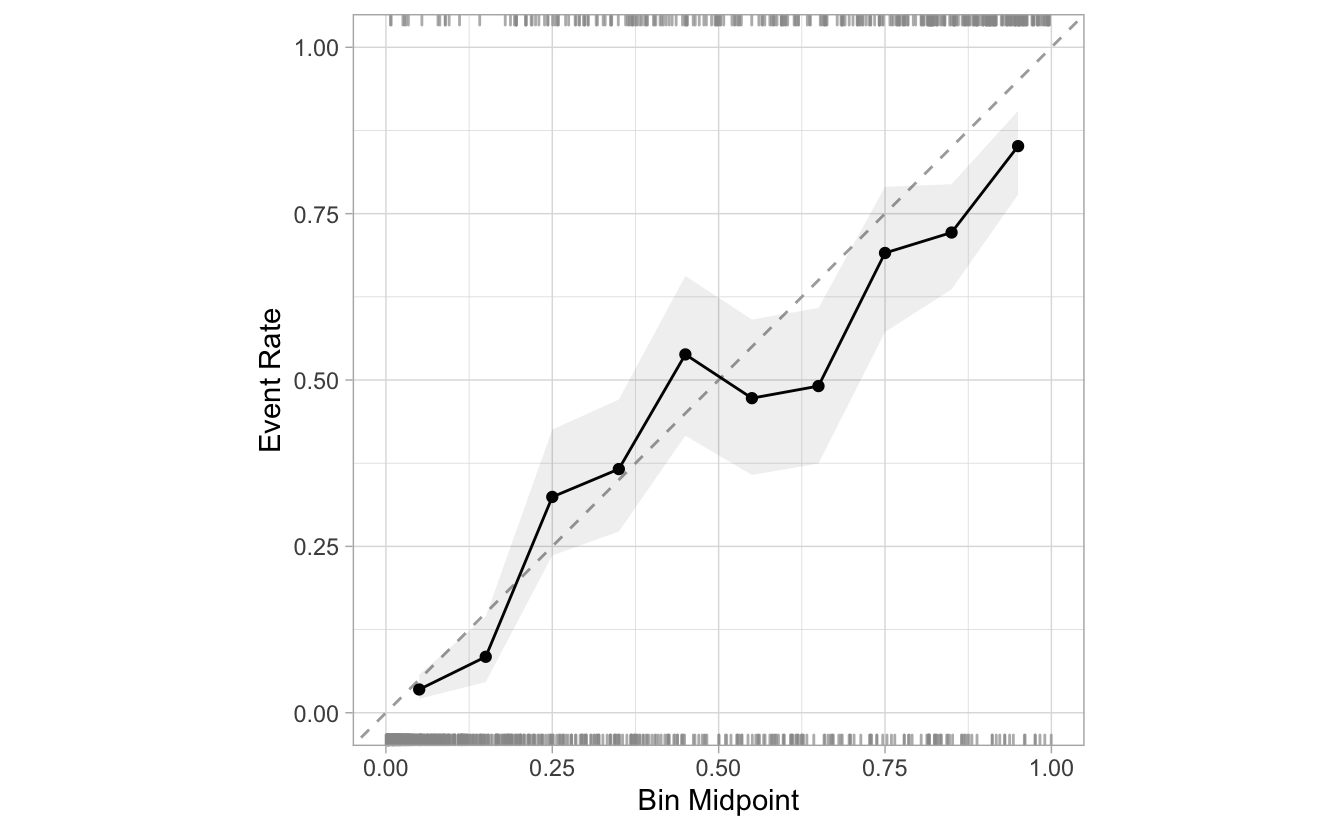The calibration plot for the ideal model will essentially be perfect incline line that start at (0,0) and ends in (1,1). In the case of this model, we can see that the seventh point has an event rate of 49.1% despite having estimated probabilities ranging from 60% to 70%. This indicates that the model is not creating predictions in this region that are consistent with the data (i.e., it is under-predicting).

The number of bins in `cal_plot_breaks()` can be adjusted using `num_breaks`. Here is an example of what the plot looks like if we reduce the bins from 10, to 5:

``````segment_logistic %>%
cal_plot_breaks(Class, .pred_good, num_breaks = 5 )
``````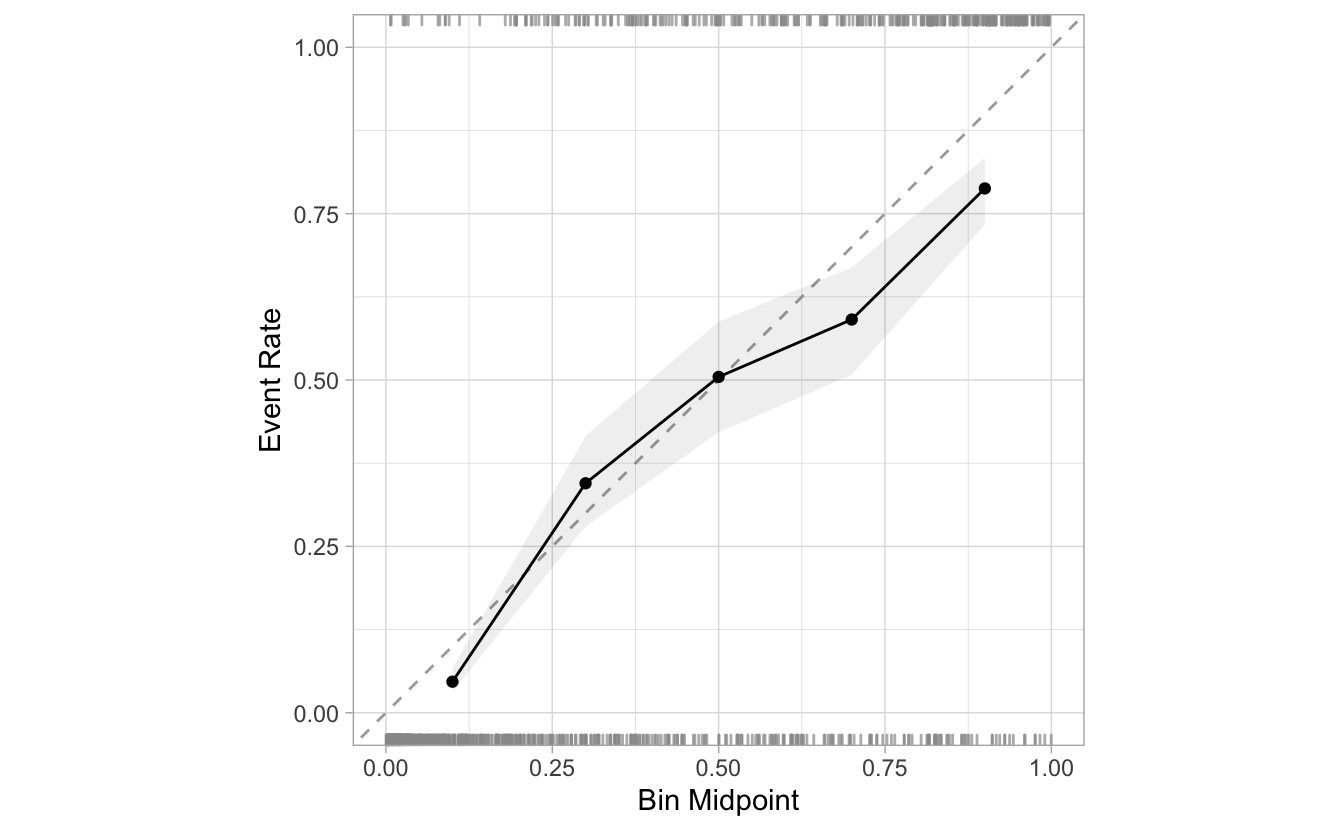The number of breaks should be based on ensuring that there is enough data in each bin to adequately estimate the observed event rate. If your data are small, the next version of the calibration plot might be a better solution.

### Windowed

Another approach is to use overlapping ranges, or windows. Like the previous plot, we bin the data and calculate the event rate. However, we can add more bins by allowing them to overlap. If the data set size is small, one strategy is to use a set of wide bins that overlap one another.

There are two variables that control the windows. The step size controls the frequency of the windows. If we set a step size of 5%, windows will be created for each 5% increment in predicted probability (5%, 10%, 15%, etc). The second argument is the (maximum) window size. If it is set to 10%—and the step size is set at 5%—then a given step will overlap halfway into both the previous step and the next step. Here is a visual representation of this specific scenario: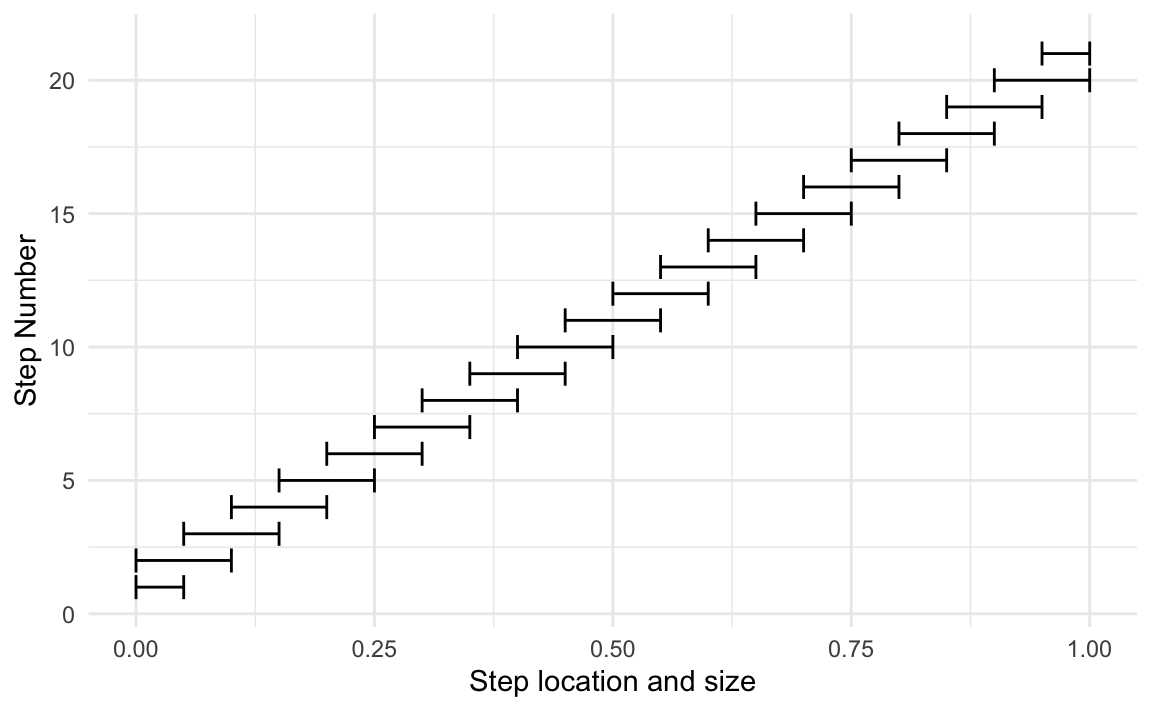In probably, the `cal_plot_windowed()` function provides this functionality. The default step size is 0.05, and can be changed via the `step_size` argument. The default window size is 0.1, and can be changed via the `window_size` argument:

``````segment_logistic %>%
cal_plot_windowed(Class, .pred_good)
``````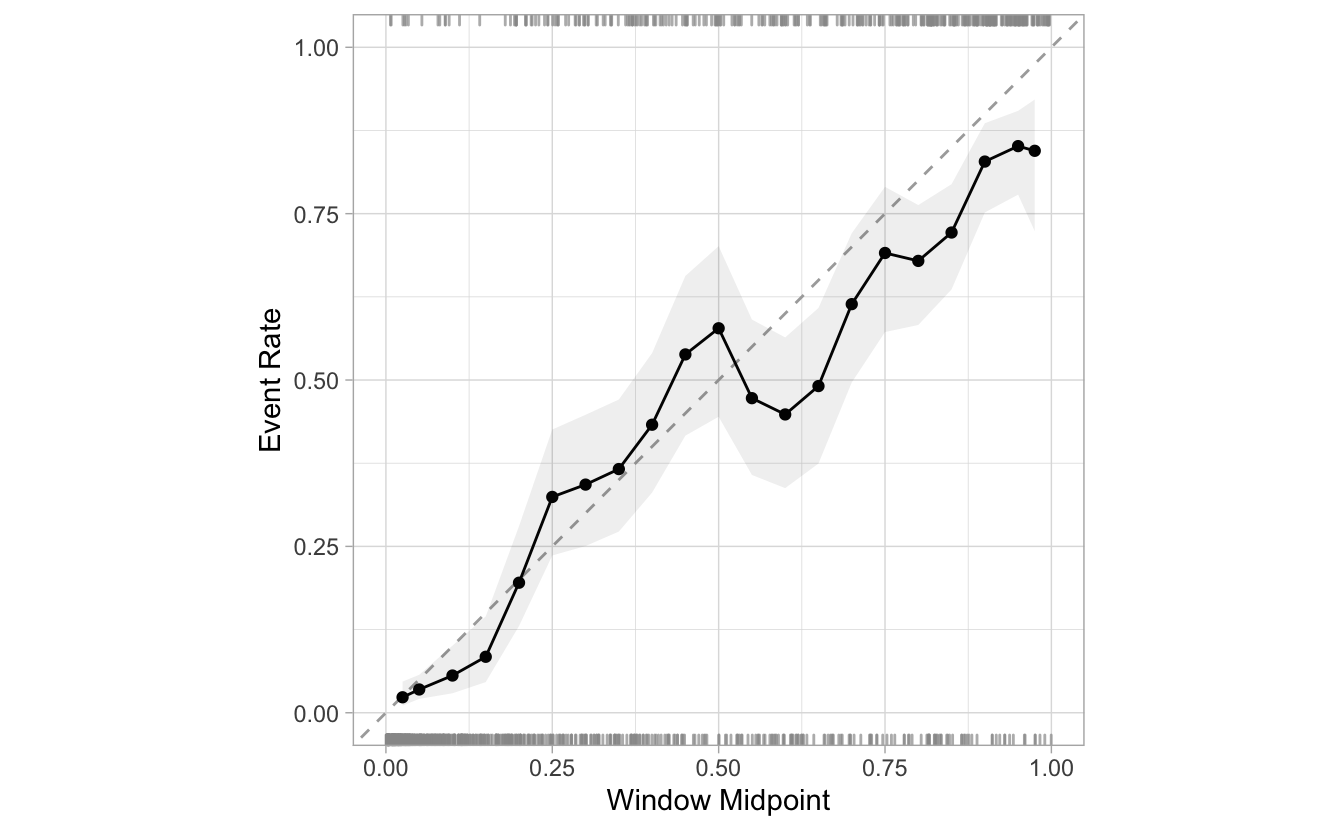Here is an example of reducing the `step_size` from 0.05 to 0.02. There are more than double the windows:

``````segment_logistic %>%
cal_plot_windowed(Class, .pred_good, step_size = 0.02)
``````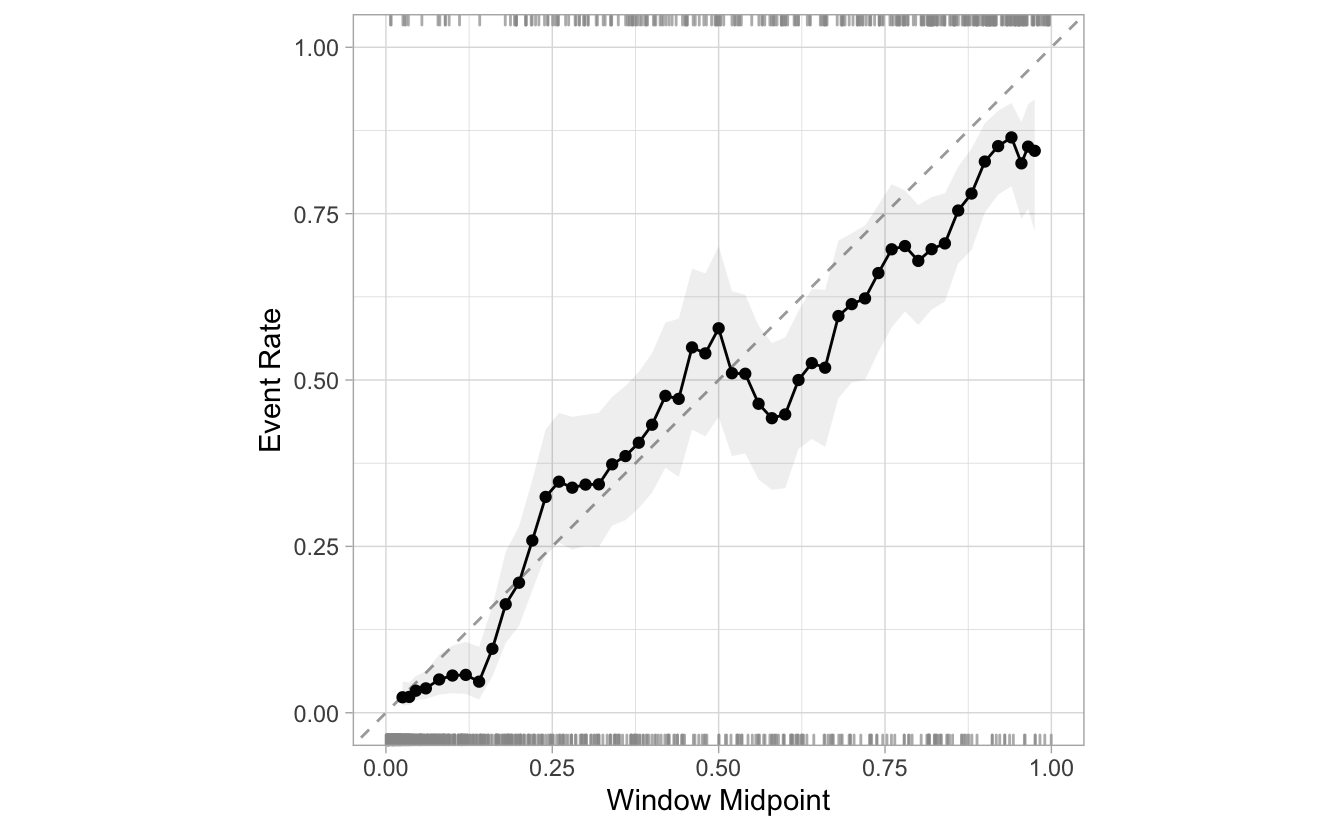### Model-Based

Another way to visualize the performance is to fit a classification model of the events against the estimated probabilities. This is helpful because it avoids the use of pre-determined groupings. Another difference is that we are not plotting midpoints of actual results, but rather predictions based on those results.

The `cal_plot_logistic()` provides this functionality. By default, it uses a logistic regression. There are two possible methods for fitting:

• `smooth = TRUE` (the default) fits a generalized additive model using splines. This allows for more flexible model fits.

• `smooth = FALSE` uses an ordinary logistic regression model with linear terms for the predictor.

As an example:

``````segment_logistic %>%
cal_plot_logistic(Class, .pred_good)
``````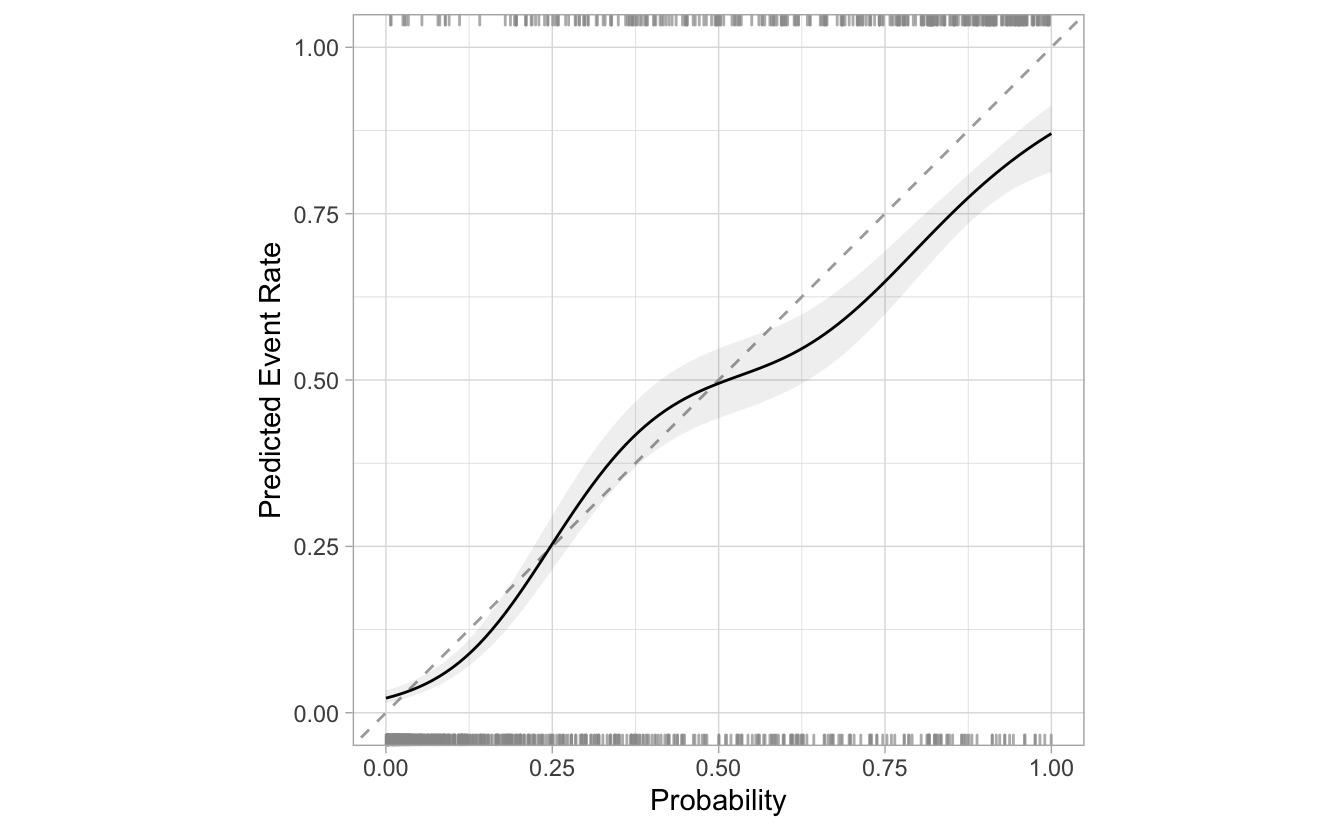The corresponding `glm()` model produces:

``````segment_logistic %>%
cal_plot_logistic(Class, .pred_good, smooth = FALSE)
``````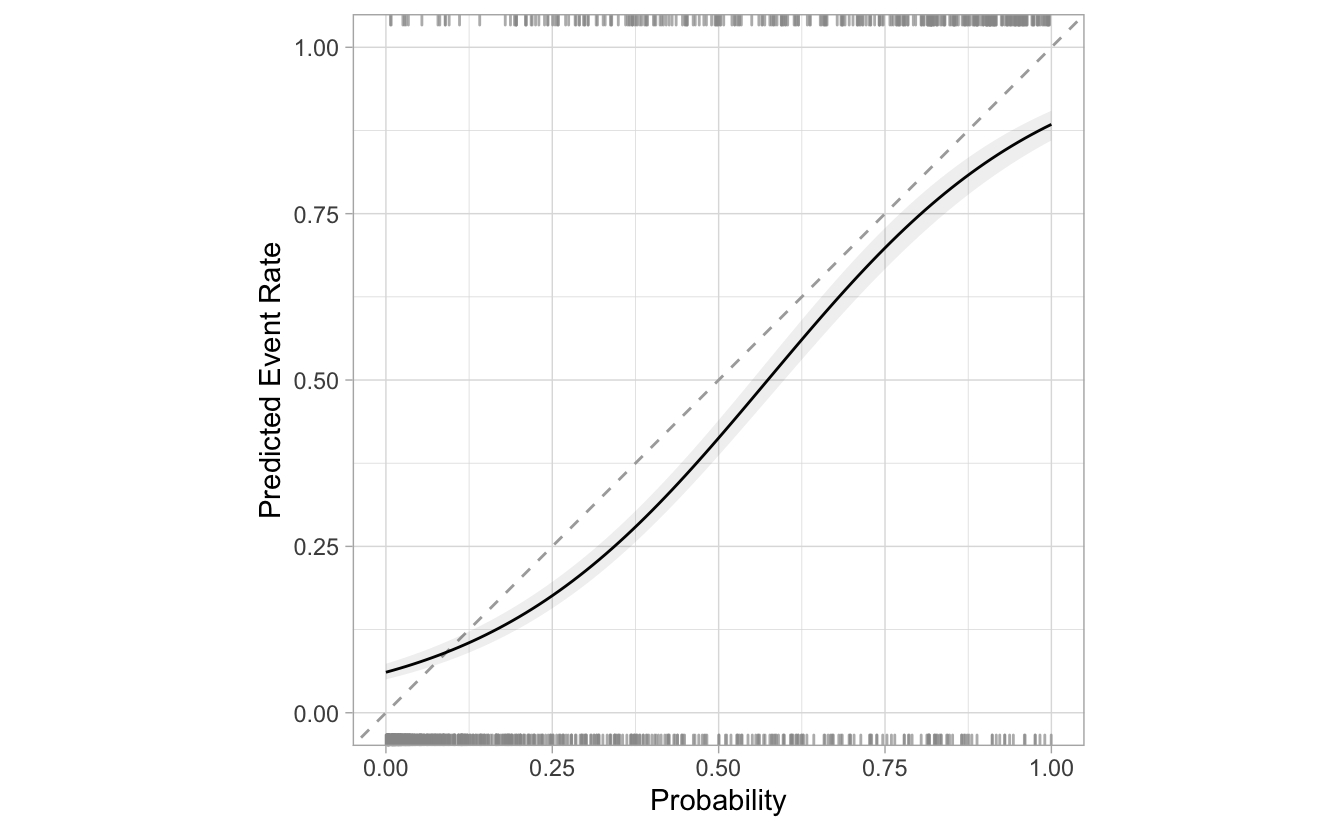#### Intervals

The confidence intervals are visualized using the gray ribbon. The default interval is 0.9, but can be changed using the `conf_level` argument.

``````segment_logistic %>%
cal_plot_breaks(Class, .pred_good, conf_level = 0.8)
``````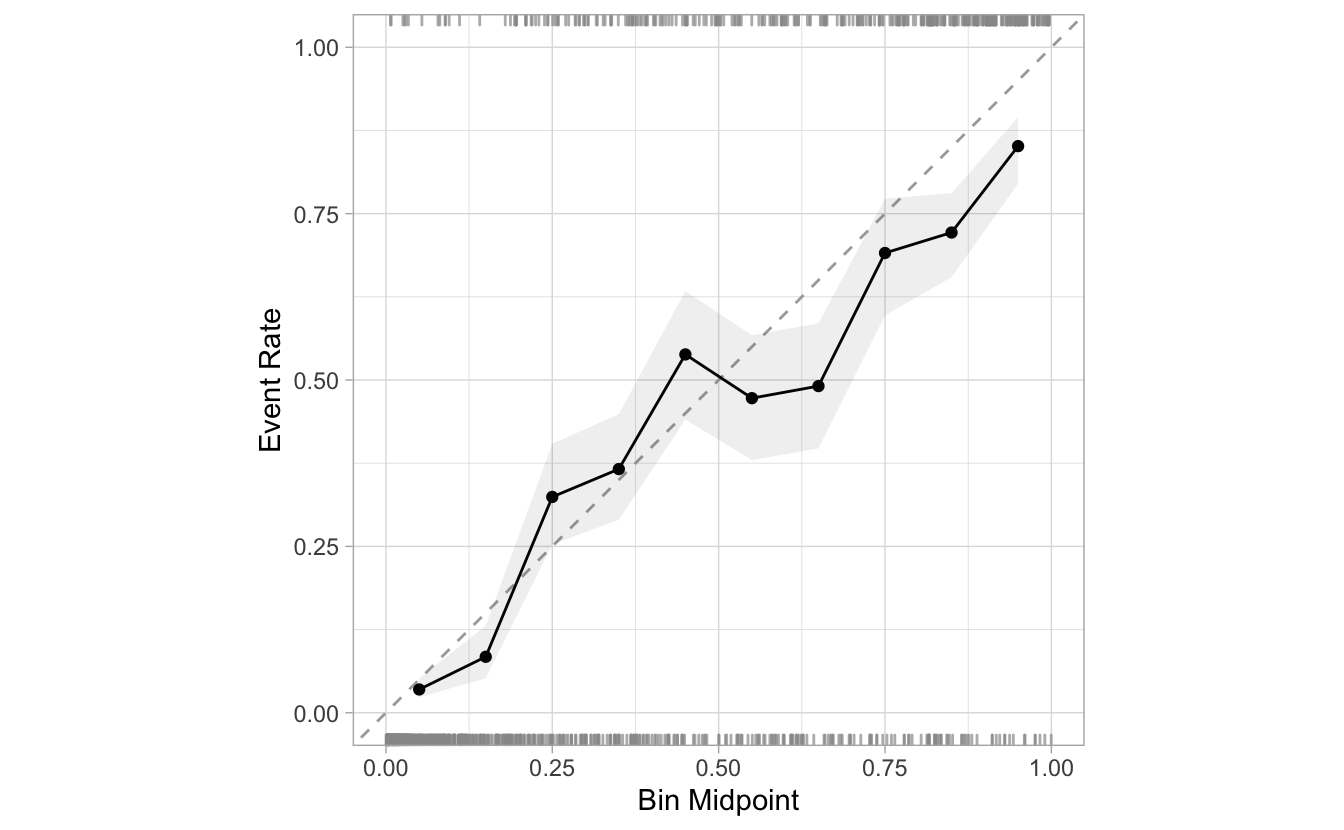If desired, the intervals can be removed by setting the `include_ribbon` argument to `FALSE`.

``````segment_logistic %>%
cal_plot_breaks(Class, .pred_good, include_ribbon = FALSE)
``````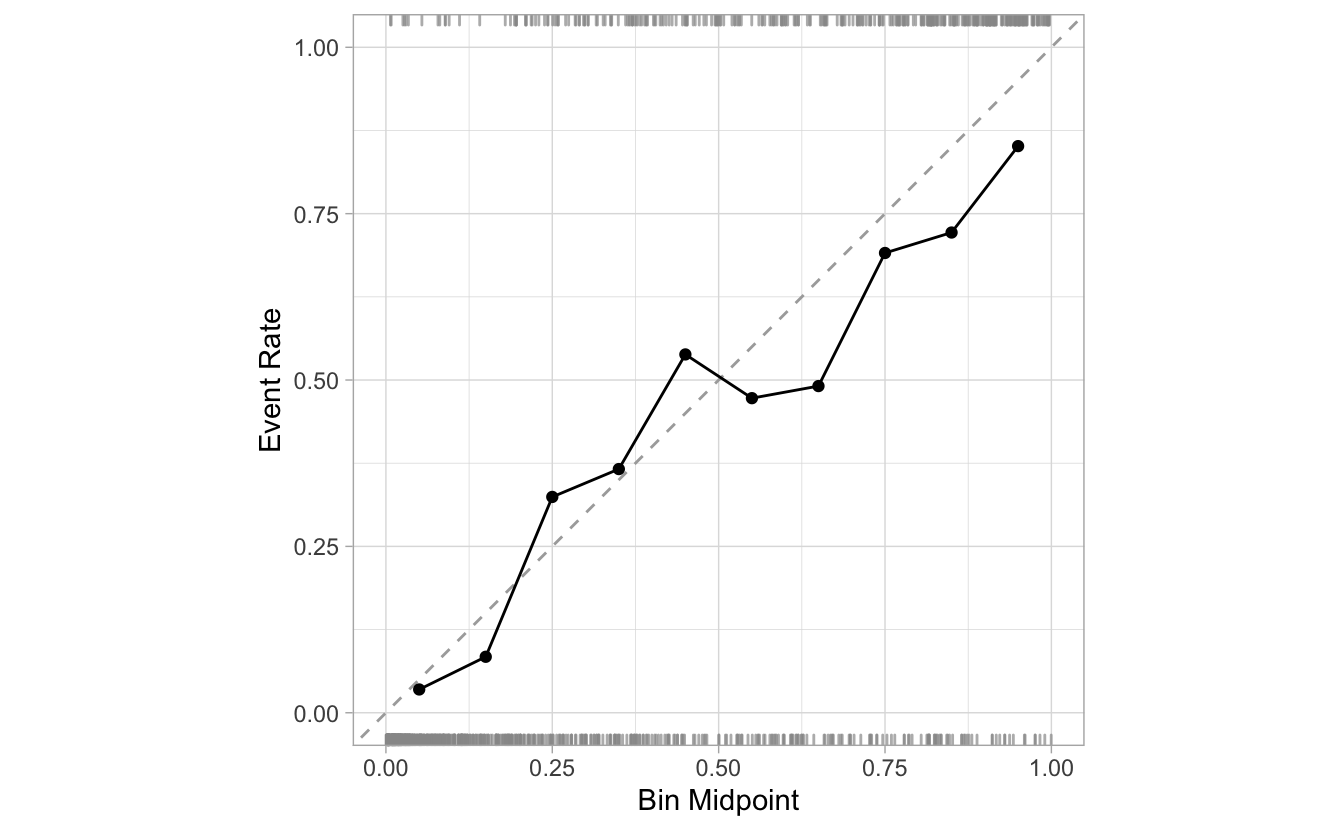#### Rugs

By default, the calibration plots include a RUGs layer at the top and at the bottom of the visualization. They are meant to give us an idea of the density of events and non-events as the probabilities progress from 0 to 1.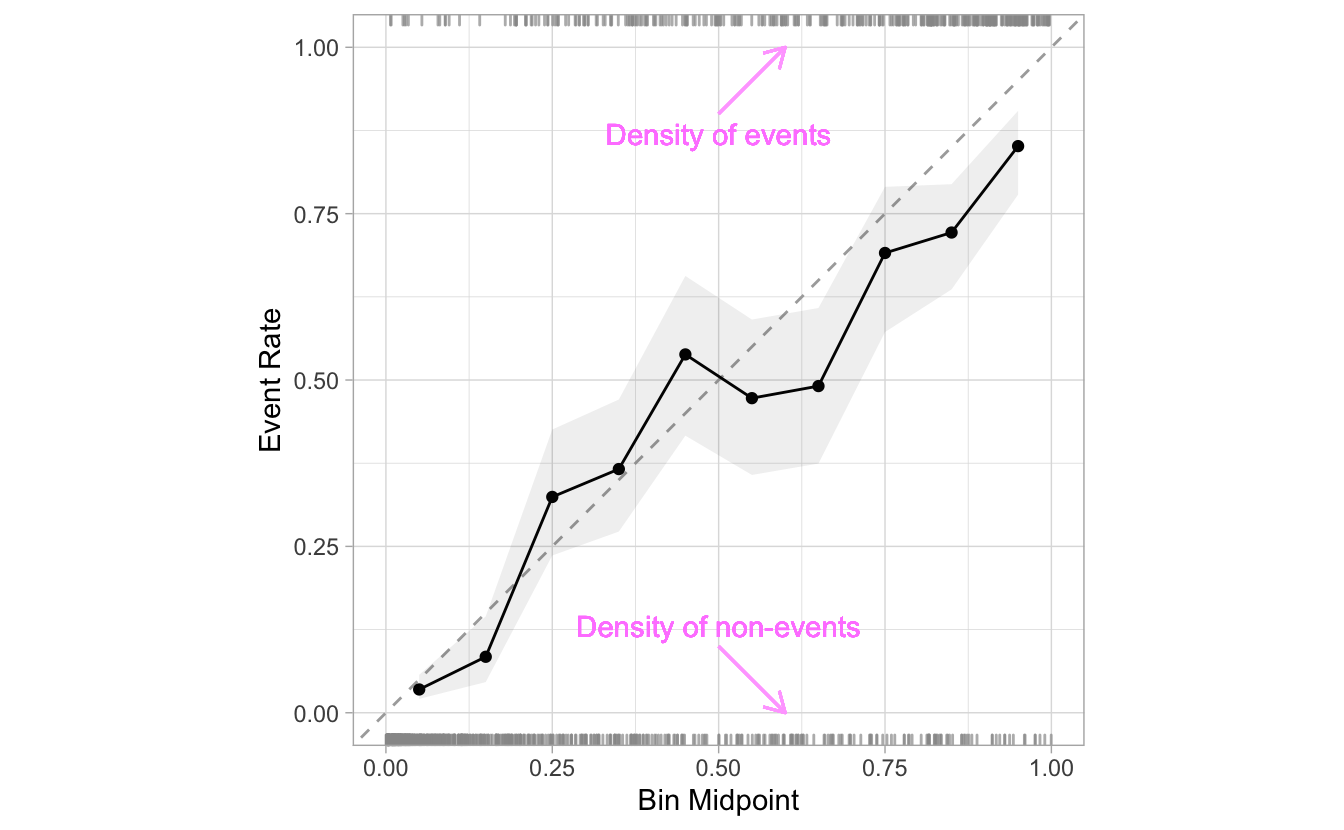This layer can be removed by setting `include_rug` to `FALSE`:

``````segment_logistic %>%
cal_plot_breaks(Class, .pred_good, include_rug = FALSE)
``````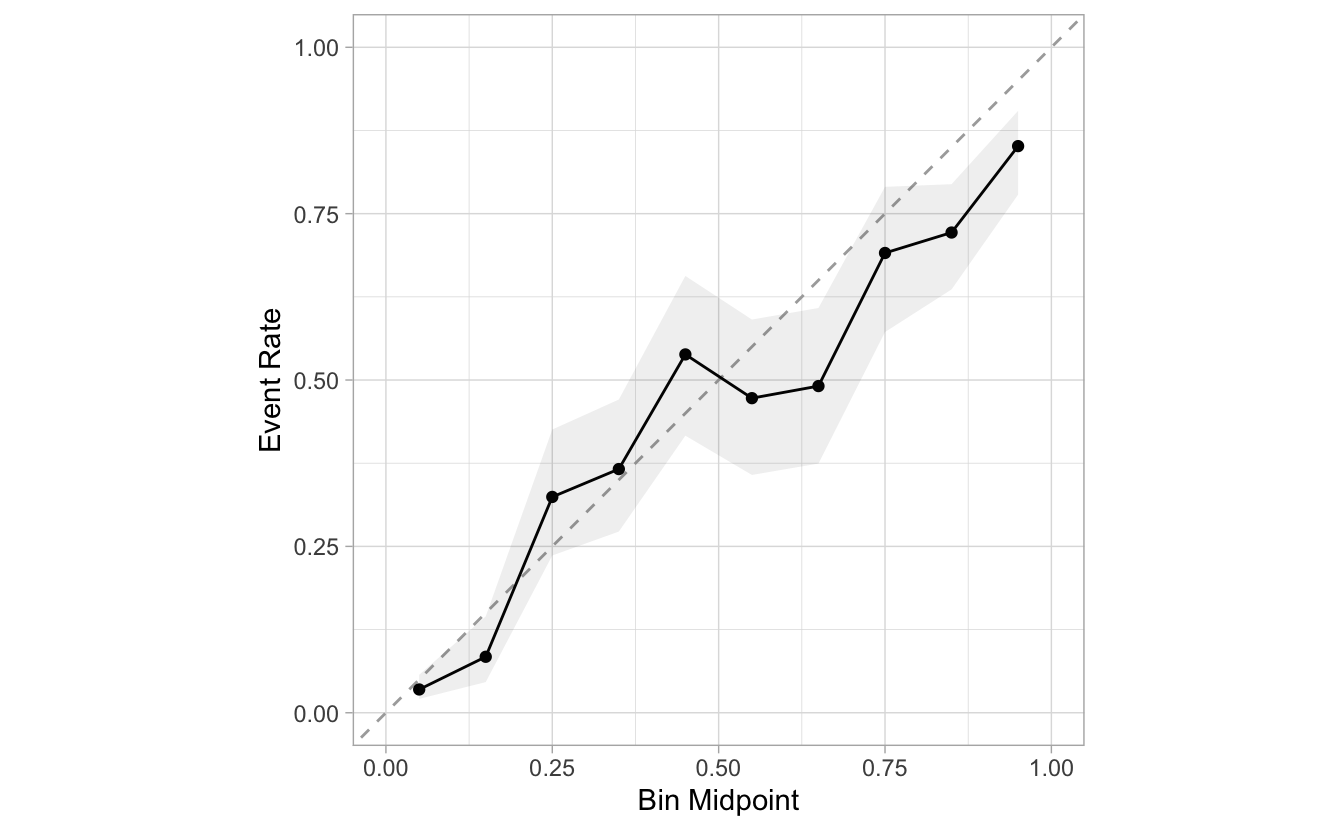## Integration with tune

So far, the inputs to the functions have been data frames. In tidymodels, the tune package has methods for resampling models as well as functions for tuning hyperparameters.

The calibration plots in the probably package also support the results of these functions (with class `tune_results`). The functions read the metadata from the tune object, and the `truth` and `estimate` arguments automatically.

To showcase this feature, we will tune a model based on simulated data. In order for the calibration plot to work, the predictions need to be collected. This is done by setting `save_pred` to `TRUE` in `tune_grid()`'s control settings.

``````set.seed(111)
sim_data <- sim_classification(500)
sim_folds <- vfold_cv(sim_data, repeats = 3)

rf_mod <- rand_forest(min_n = tune()) %>% set_mode("classification")

set.seed(222)
tuned_model <-
rf_mod %>%
tune_grid(
class ~ .,
resamples = sim_folds,
grid = 4,
# Important: `saved_pred` has to be set to TRUE in order for
# the plotting to be possible
control = control_resamples(save_pred = TRUE)
)

tuned_model
#> # Tuning results
#> # 10-fold cross-validation repeated 3 times
#> # A tibble: 30 × 6
#>    splits           id      id2    .metrics         .notes           .predicti…¹
#>    <list>           <chr>   <chr>  <list>           <list>           <list>
#>  1 <split [450/50]> Repeat1 Fold01 <tibble [8 × 5]> <tibble [0 × 3]> <tibble>
#>  2 <split [450/50]> Repeat1 Fold02 <tibble [8 × 5]> <tibble [0 × 3]> <tibble>
#>  3 <split [450/50]> Repeat1 Fold03 <tibble [8 × 5]> <tibble [0 × 3]> <tibble>
#>  4 <split [450/50]> Repeat1 Fold04 <tibble [8 × 5]> <tibble [0 × 3]> <tibble>
#>  5 <split [450/50]> Repeat1 Fold05 <tibble [8 × 5]> <tibble [0 × 3]> <tibble>
#>  6 <split [450/50]> Repeat1 Fold06 <tibble [8 × 5]> <tibble [0 × 3]> <tibble>
#>  7 <split [450/50]> Repeat1 Fold07 <tibble [8 × 5]> <tibble [0 × 3]> <tibble>
#>  8 <split [450/50]> Repeat1 Fold08 <tibble [8 × 5]> <tibble [0 × 3]> <tibble>
#>  9 <split [450/50]> Repeat1 Fold09 <tibble [8 × 5]> <tibble [0 × 3]> <tibble>
#> 10 <split [450/50]> Repeat1 Fold10 <tibble [8 × 5]> <tibble [0 × 3]> <tibble>
#> # … with 20 more rows, and abbreviated variable name ¹​.predictions``````

The plotting functions will automatically collect the predictions. Each of the pre-processing groups will be plotted individually in its own facet.

``````tuned_model %>%
cal_plot_logistic()
``````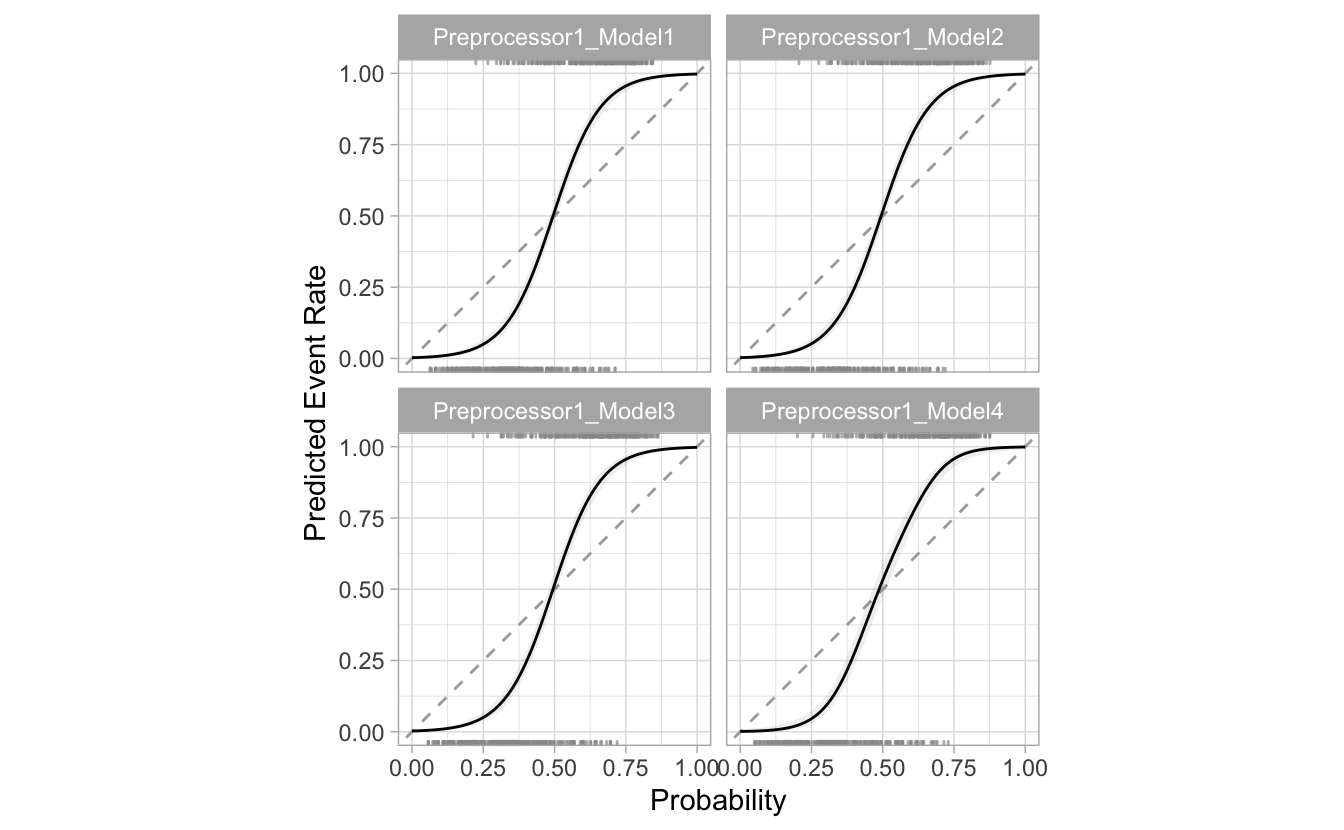A panel is produced for each value of `min_n`, coded with an automatically generated configuration name. This makes sure to use the out-of-sample data to make the plot (instead of just re-predicting the training set).

## Preparing for the next stage

As mentioned in the outset of this post, the goal is to also provide a way to calibrate the model, and to apply the calibration to future predictions. We have made sure that the plotting functions are ready now to accept multiple probability sets.

In this post, we will showcase that functionality by “manually” creating a quick calibration model and comparing its output to the original probabilities. We will need both of them in the same data frame, as well as a variable distinguishing the original probabilities from the calibrated probabilities. In this case we will create a variable called `source`:

``````model <- glm(Class ~ .pred_good, segment_logistic, family = "binomial")

preds <- predict(model, segment_logistic, type = "response")

combined <- bind_rows(
mutate(segment_logistic, source = "original"),
mutate(segment_logistic, .pred_good = 1 - preds, source = "glm")
)

combined
#> # A tibble: 2,020 × 4
#>    .pred_poor .pred_good Class source
#>         <dbl>      <dbl> <fct> <chr>
#>  1    0.986      0.0142  poor  original
#>  2    0.897      0.103   poor  original
#>  3    0.118      0.882   good  original
#>  4    0.102      0.898   good  original
#>  5    0.991      0.00914 poor  original
#>  6    0.633      0.367   good  original
#>  7    0.770      0.230   good  original
#>  8    0.00842    0.992   good  original
#>  9    0.995      0.00458 poor  original
#> 10    0.765      0.235   poor  original
#> # … with 2,010 more rows``````

The new plot functions support dplyr groupings. So, to overlay the two groups, we just need to pass `source` to `group_by()`:

``````combined %>%
group_by(source) %>%
cal_plot_breaks(Class, .pred_good)
``````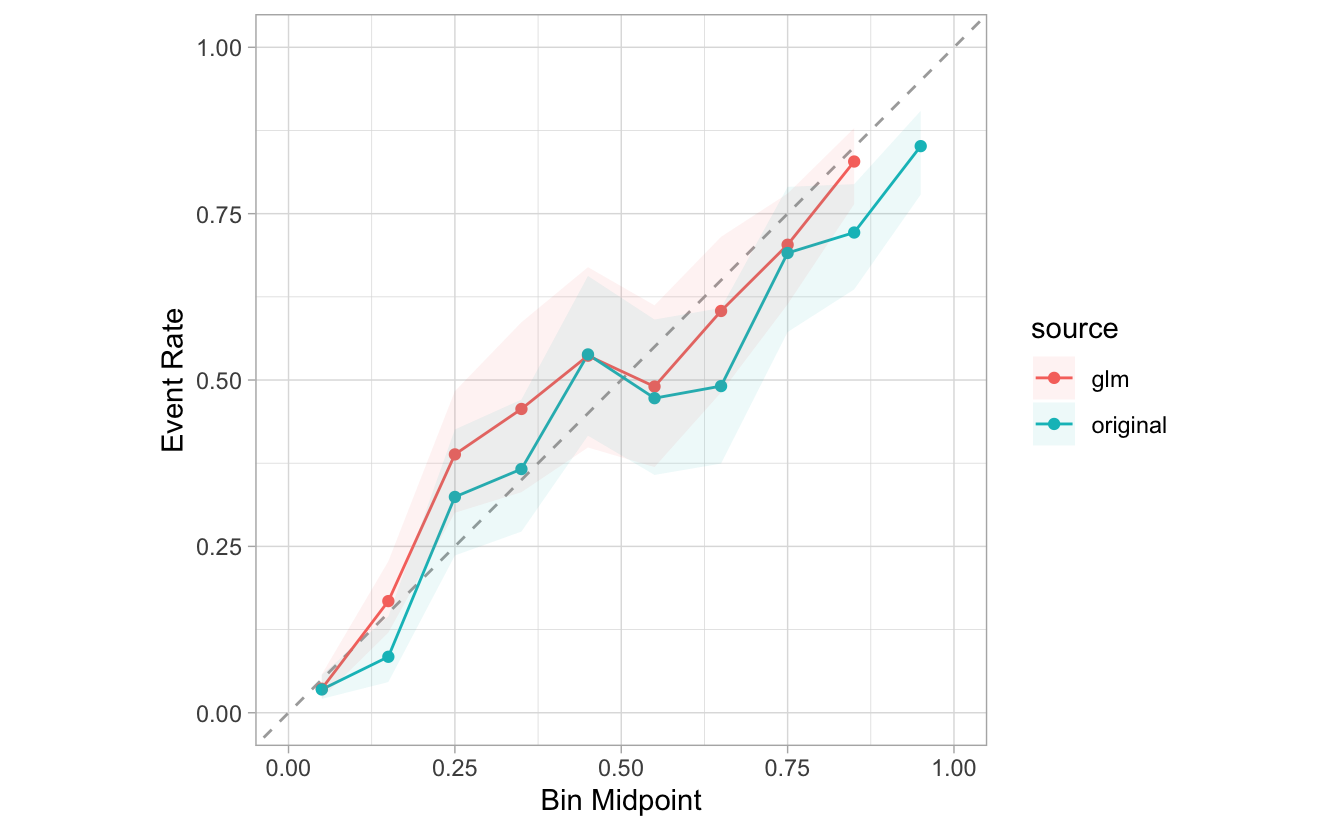If we would like to plot them side by side, we can add `facet_wrap()` as an additional step of the plot:

``````combined %>%
group_by(source) %>%
cal_plot_breaks(Class, .pred_good) +
facet_wrap(~source) +
theme(legend.position = "none")
``````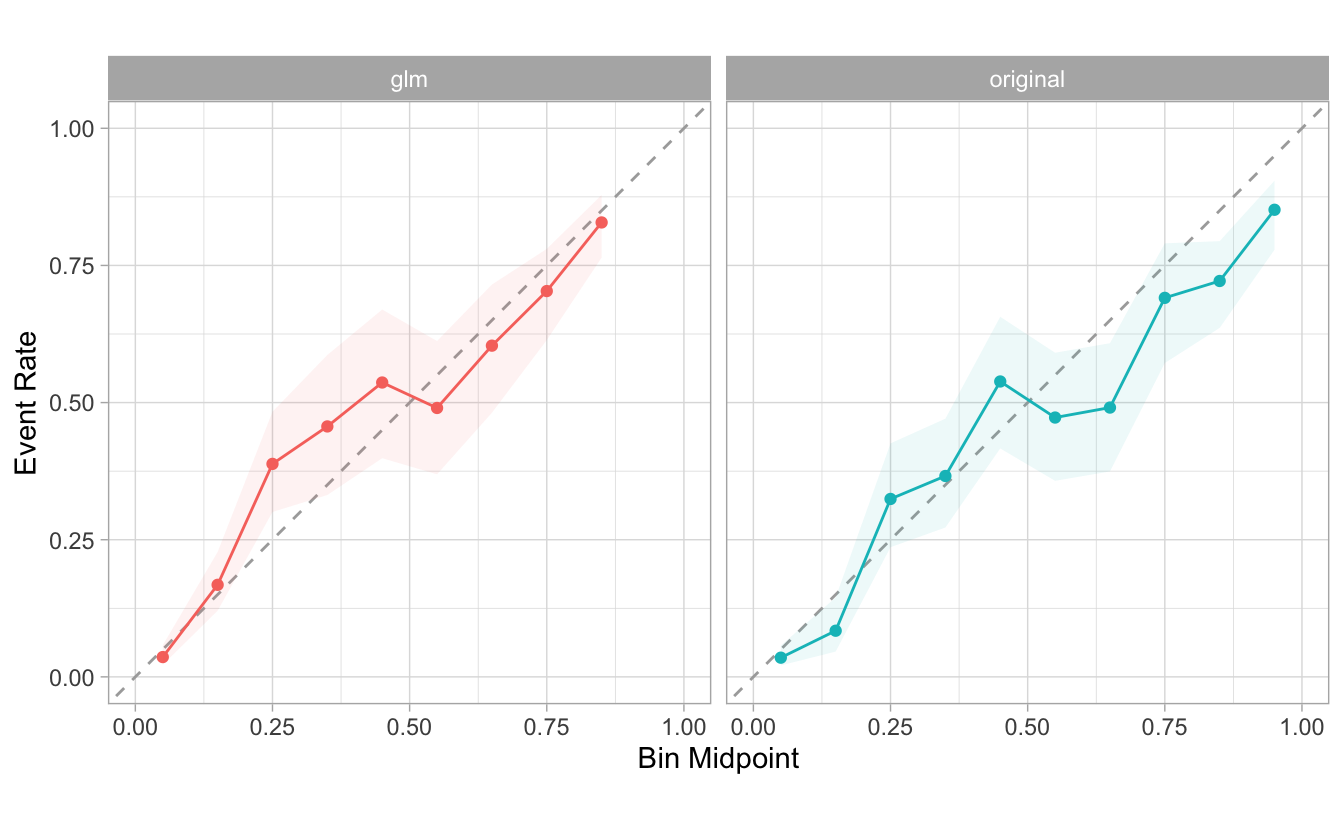Our goal in the future is to provide calibration functions that create the models, and provide an easy way to visualize them.

## Conclusion

As mentioned at the top of this post, we welcome your feedback as you try out these features and read about our plans for the future. If you wish to send us your thoughts, feel free to open an issue in probably’s GitHub repo here: https://github.com/tidymodels/probably/issues.

1. We can think of an event as the outcome that is being tracked by the probability. For example, if a model predicts “heads” or “tails” and we want to calibrate the probability for “tails”, then the event is when the column containing the outcome, has the value of “tails”. ↩︎

Contents